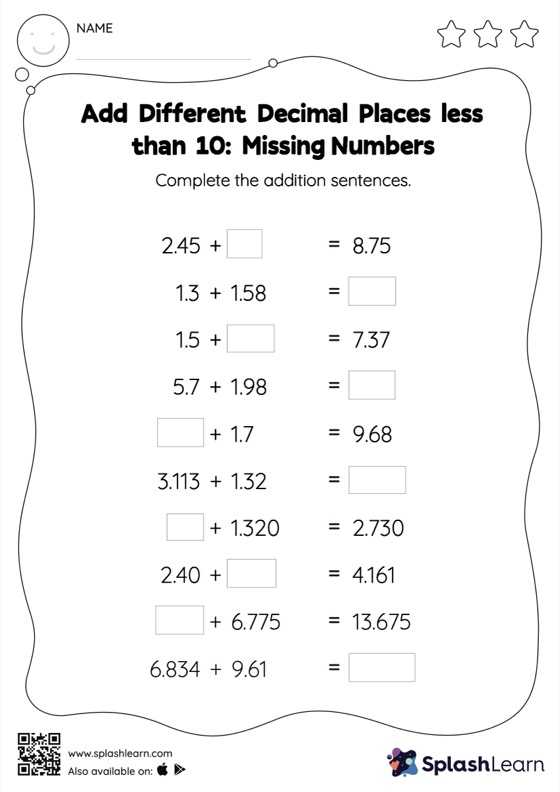# Add Different Decimal Places less than 10: Missing Numbers Worksheet

Home > Add Different Decimal Places less than 10: Missing NumbersTask your little mathematicians to crack the code of adding different decimal places less than 10 with this fun worksheet. When adding decimals on this worksheet, students align the decimal points and use zero as a placeholder. Then to find the missing number in the add different decimal places less than 10 worksheet, students then apply the relationship between addition and subtraction.